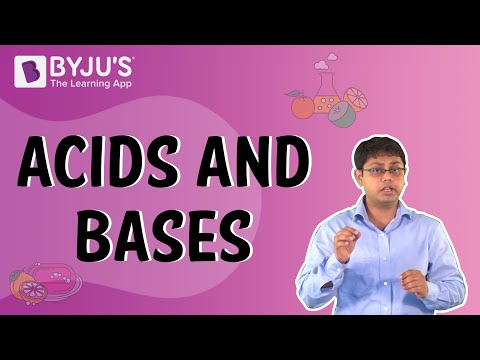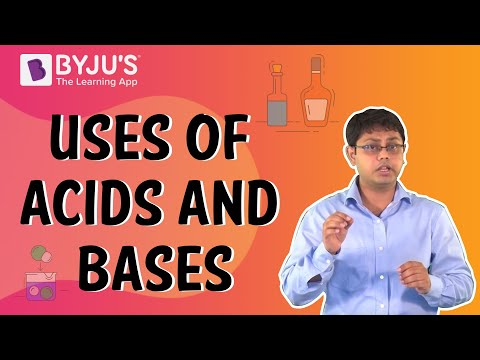# Acids and Bases Questions

Acids and bases are important in chemistry. We encounter them in our daily lives, from cleaning agents such as soaps and detergents to baking soda. Corrosive substances are classified into two types: acids and bases (alkaline earth metal chemical elements). Any compound with a pH value between zero and seven is considered acidic, while any compound with a pH value between seven and fourteen is considered base. An acid is referred to as a proton donor, whereas a base is referred to as a proton acceptor.

 Definition: The sour taste of acidic substances is usually used to identify them. An acid is essentially a molecule that can donate an H+ ion while remaining energetically favourable after losing H+. Blue litmus paper is known to turn red when exposed to acids. Bases, on the other hand, have a bitter flavour and a slippery texture. An alkali is a base that can be dissolved in water. When these substances react chemically with acids, salts are formed. Bases have been observed to turn red litmus blue.

## Acids and Bases Chemistry Questions with Solutions

Q1. Which one of the following is the strongest acid?

a) CH2ClCOOH

b) CH3COOH

c) CHCl2COOH

d) CCl3COOH

Explanation. Strong acid means weak conjugate base. CCl3COO < CHCl2COO < CH2ClCOO < CH3COO. Therefore, the strongest acid is CCl3COOH.

Q2. Lime water is-

a) CaO

b) Ca(OH)2

c) CaCO3

d) CaCI2

Explanation. A saturated aqueous solution of calcium hydroxide is known as limewater.

Q3. The chemical formula of caustic potash is

a) NaOH

b) Ca(OH)2

c) NH4OH

d) KOH

Explanation. Potassium hydroxide, also known as caustic potash, is an inorganic compound with the formula KOH.

Q4. Bleaching powder gives the smell of chlorine because it-

a) is unstable.

b) gives chlorine on exposure to the atmosphere.

c) is a mixture of chlorine and slaked lime.

d) contains an excess of chlorine.

Correct Answer. (d) contains an excess of chlorine.

Q5. Arrange these acids in order of increasing strength.

acid A: pKa = 1.52

acid B: pKa = 6.93

acid C: pKa = 3.86

Given solutions with the same initial concentration of each acid, which would have the highest percent ionization?

Answer. Acids are listed in ascending order of strength: acid B < acid C < acid A. Acid A has the highest percent of ionisation given the same initial concentration of each acid because it is the strongest acid.

Q6. Arrange these bases in order of increasing strength:

base A: pKb = 13.10

base B: pKb = 8.74

base C: pKb = 11.87

Given solutions with the same initial concentration of each base, which would have the highest percent ionization?

Answer. Bases are listed in ascending order of strength: base A < base C < base B. Since base A is the weakest base, it has the highest percent of ionisation in solutions with the same initial concentration of each base.

Q7. (a) Write the name given to bases that are highly soluble in water. Give an example.

(b) How is tooth decay related to pH? How can it be prevented?

(c) Why does bee sting cause pain and irritation? Rubbing of baking soda on the sting area gives relief. How?

Answer. (a) Alkali, such as NaOH (Sodium hydroxide).

(b) A lower pH in the mouth promotes the growth of bad bacteria, which eventually leads to tooth decay.

It is preventable by avoiding foods that cause acidity.

(c) It is caused by formic acid. Formic acid is neutralised by sodium hydrogen carbonate (baking soda), giving relief.

Q8. “Sodium hydrogen carbonate is a basic salt”. Justify the statement. How is it converted into washing soda? Explain.

Answer. Sodium hydrogen carbonate is a salt formed by the reaction of sodium hydroxide (a strong base) and carbonic acid (weak acid). It is a basic salt. Heating and crystallisation are used to convert it to washing soda.

2NaHCO3 → Na2CO3 → CO2 +H2O.

Q9. What are the conjugate bases of the Bronsted acids HF, H2SO4, and HCO3?

Answer. The conjugate bases for the Bronsted acids are F, HSO4, and CO32–.

Q10. Why do 1 M HCl solutions have a higher concentration of H+ ions than 1M CH3COOH solution?

Answer. HCl is more strong than CH3COOH. There is complete dissociation of HCl, which produces more H+ ions than CH3COOH, a weak acid. The concentration is determined by the presence of H+ ions. As a result, 1 M HCL has a greater concentration than 1 M CH3COOH.

Q11. How is the concentration of hydroxide ions(OH) affected when excess base is dissolved in a solution of sodium hydroxide?

Answer. When a base is dissolved in a sodium hydroxide solution, the concentration of OH ions per unit volume in the solution increases.

Q12. Give Arrhenius’s definition of an acid and a base.

Answer. Acids are defined by Arrhenius as substances that release H+ ions when dissolved in water.

Bases are substances that, when dissolved in water, release OH ions.

Q13. What do you mean by the water of crystallization of a substance? Describe an activity to show that blue copper sulphate crystals contain water of crystallization.

Answer. The fixed number of water molecules present in one formula unit of salt in its crystalline form is referred to as water of crystallisation.

CuSO4.5H2O is an example.

It means that one formula unit of copper sulphate contains five water molecules.

Activity to demonstrate the crystallisation of water:

1) Place a few copper sulphate crystals in a dry test tube and heat it.

2) We see water droplets on the test tube walls, and the salt turns white.

3) Place 2 – 3 drops of water on the heated copper sulphate sample.

4) We notice that the blue colour of copper sulphate crystals has been restored.

Reason:

1) In the above activity, copper sulphate crystals that appear to be dry contain water of crystallisation; when heated, the water present in the crystals evaporates and the salt turns white.

2) The blue colour returns when the crystals are moistened with water.

Q14. State the reason for the following statements:

(i) Tap water conducts electricity whereas distilled water does not.

(ii) Dry hydrogen chloride gas does not turn blue litmus red whereas dilute hydrochloric acid does.

(iii) During the summer season, a milkman usually adds a very small amount of baking soda to fresh milk.

(iv) For a dilution of acid, acid is added into water and not water into acid.

(v) Ammonia is a base but does not contain a hydroxyl group.

(i) Tap water contains ions that conduct electricity, whereas distilled water does not.

(ii)While dry HCl does not form ions, it does produce H+ and Cl.

(iii) Baking soda prevents milk from converting to lactic acid, which causes milk to sour.

(iv) Adding water to acid produces a strong exothermic reaction. As a result, water is slowly added to acid while it cools.

(v) Because ammonia dissolves in water and forms H, it is basic in nature.

Q15. (i) Explain why is hydrochloric acid a strong acid and acetic acid, is a weak acid. How can it be verified?

(ii) Explain why the aqueous solution of an acid conducts electricity.

(iii) You have four solutions A, B, C and D. The pH of solution A is 6, B is 9, C is 12 and D is 7,

(a) Identify the most acidic and most basic solutions.

(b) Arrange the above four solutions in the increasing order of H+ ion concentration.

(c) State the change in colour of pH paper on dipping in solutions C and D.

(i) In aqueous solution, HCl is completely ionised, whereas acetic acid is partially ionised. With pH paper, HCl produces a dark red colour, whereas CH3COOH produces an orange colour

(ii) This is due to acid ionisation in an aqueous solution, and these ions conduct electricity. (Hi) (iii)

(a) ‘A’ is the most acidic, while ‘C’ is the most basic.

(b) C (10–12) < B (10–9) < D (10–7) < A (10–6)

(c) pH paper turns blue in ‘C’ and green in ‘D.’

## Practise Questions on Acids and Bases

Q1. Methyl orange is-

(a) Pink in acidic medium, yellow in basic medium

(b) Yellow in acidic medium, pink in basic medium

(c) Colourless in acidic medium, pink in basic medium

(d) Pink in acidic medium, colourless in basic medium.

Q2. How is the concentration of hydronium (H3O+) ions affected when a solution of an acid is diluted?

Q3. Why does dry HCl gas not change the colour of the dry litmus paper?

Q4. A white powder is added while baking bread and cakes to make them soft and fluffy. Write the name of the powder. Name its main ingredients. Explain the function of each ingredient. Write the chemical reaction taking place when the powder is heated during baking.

Q5. (a) Identify the compound of calcium which is yellowish-white powder and is used for disinfecting drinking water. Write its chemical name and formula. How is it manufactured? Write the chemical equation for the reaction involved. Also, list two other uses of the compound.

(b) Write the balanced chemical equation of chlor – alkali process.

Click the PDF to check the answers for Practice Questions.

Click the PDF to check the answers for Practice Questions.

## Recommended Videos

#### Acids and Bases#### Uses of Acids and Bases#### Neutralization Reaction